# Minimax Algorithm in Chess, Checkers & Tic-Tac-Toe## Minimax Algorithm Definition

The minimax algorithm is a decision rule that minimizes the maximum possible loss of a worst-case scenario for the player. The minimax algorithm is able to minimize potential loss by using positional evaluations to predict the opponent's next move. The results of a minimax algorithm are normally displayed on a tree diagram to represent each combination.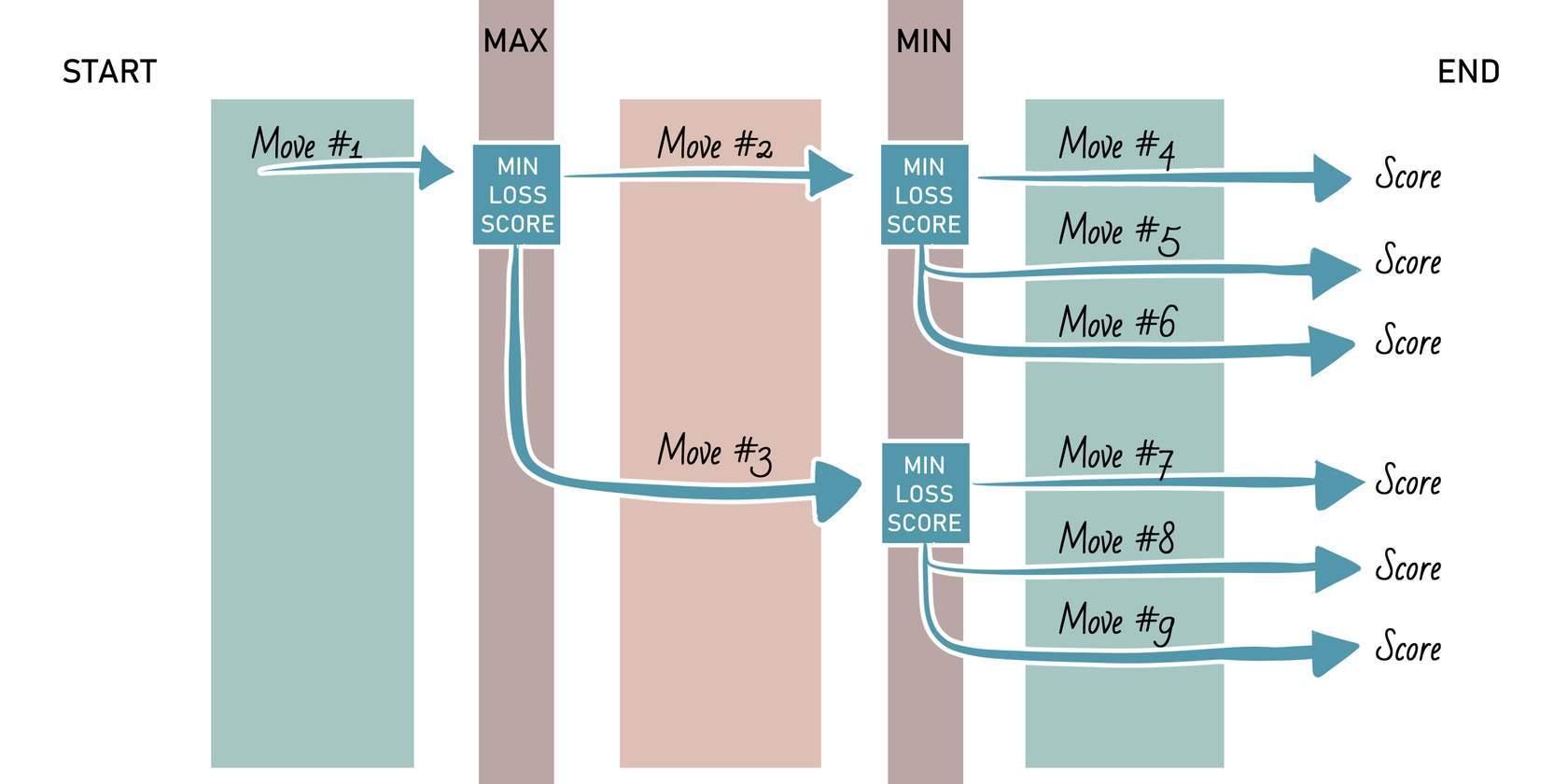## Minimax Algorithm Process in Chess

### Step 1: Create the Tree Diagram

Start by creating a tree diagram that shows all possible moves. Each move should be drawn on an arrow to represent the player choosing that move, and it should point to a circle that represents the score of the combination that minimizes possible loss.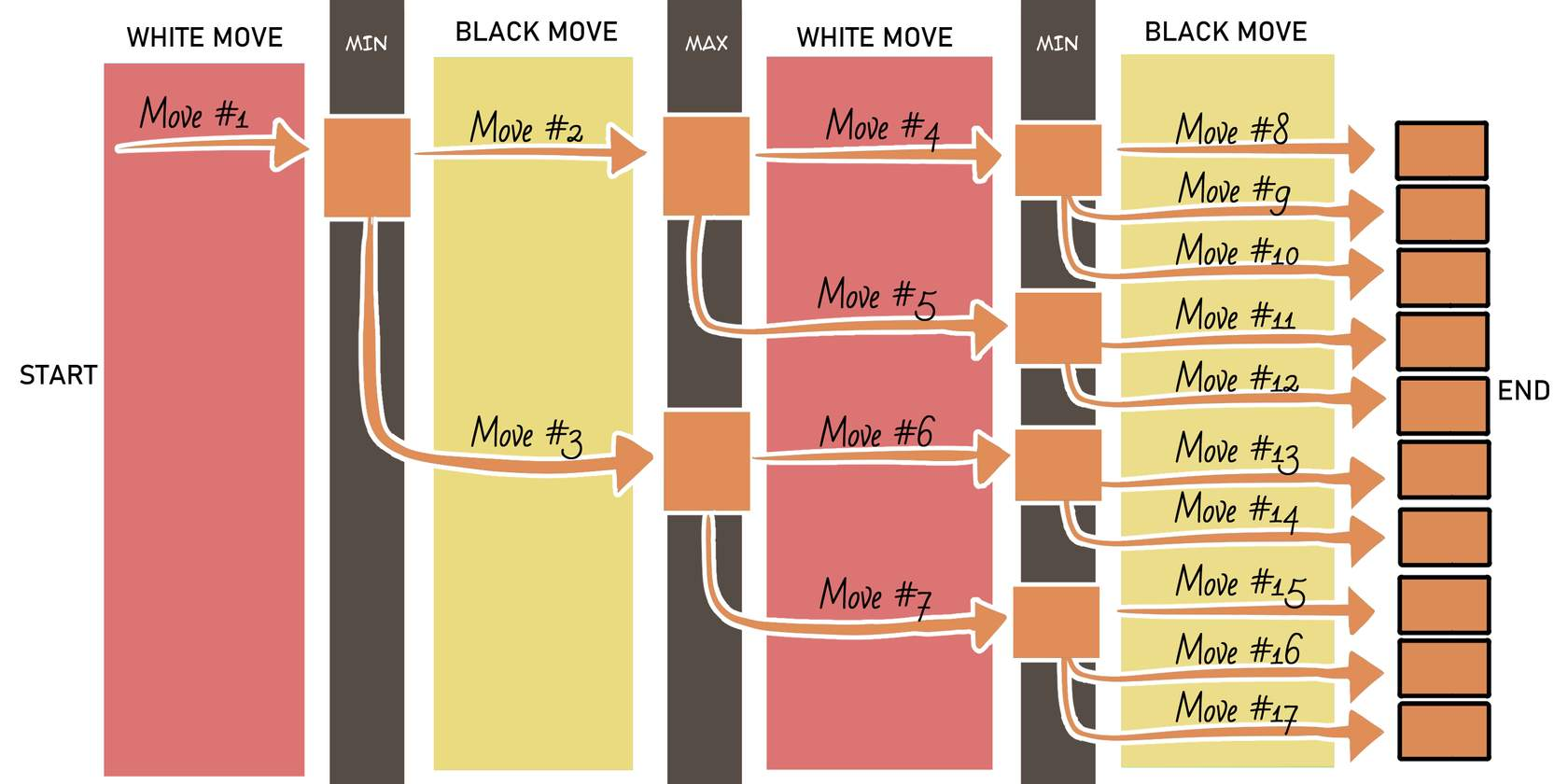### Step 2: Evaluate the Final Positions

Next, at the end circles of the tree diagram, evaluate what the board score would be if the players chose that combination of moves.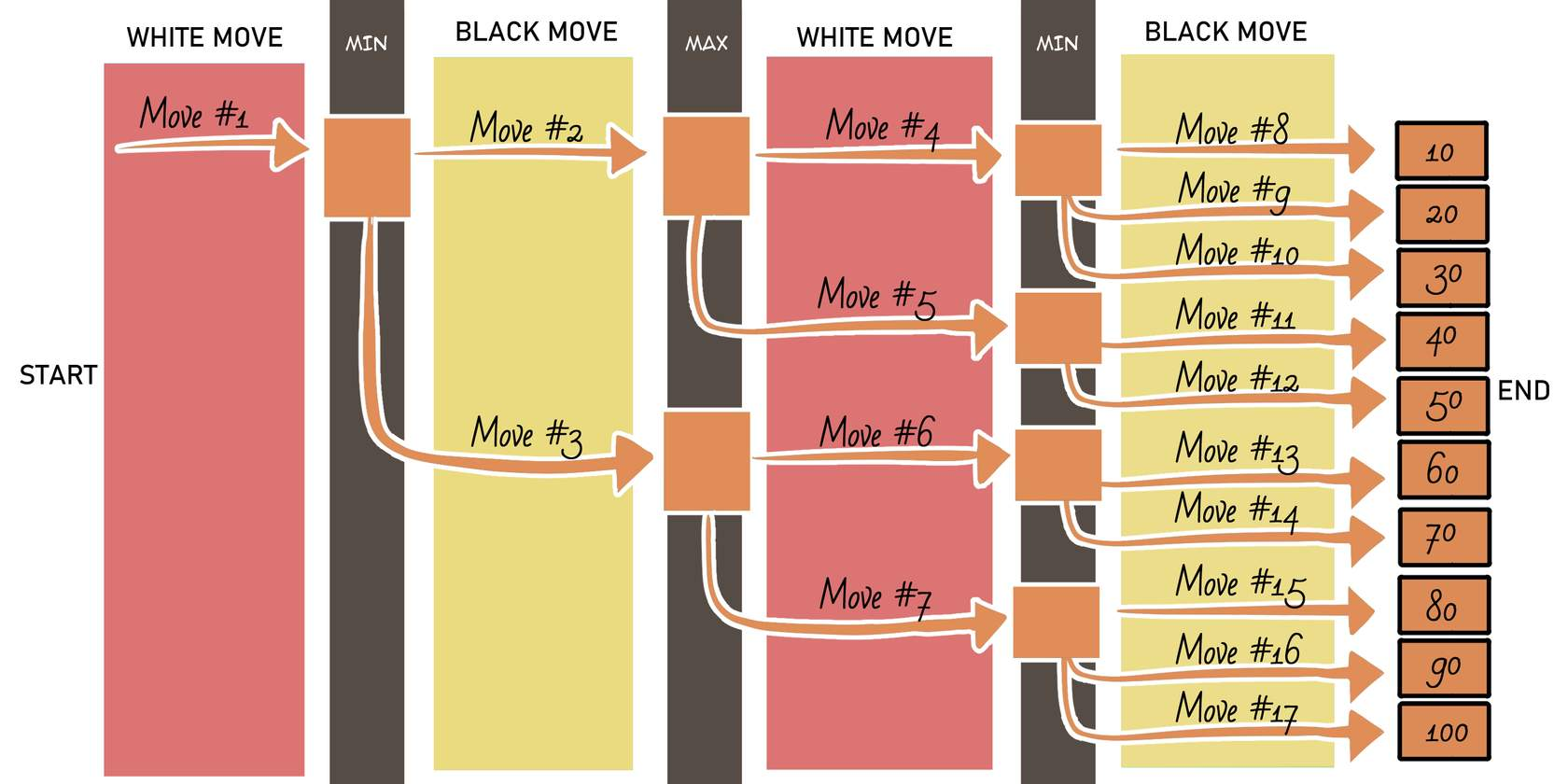### Step 3: Minimize the Potential Score

Since the player with black pieces has the last move, find the lowest score out of each group of moves with arrows from the same prior move, and place that score in the circle of the prior move to represent its worst-case scenario.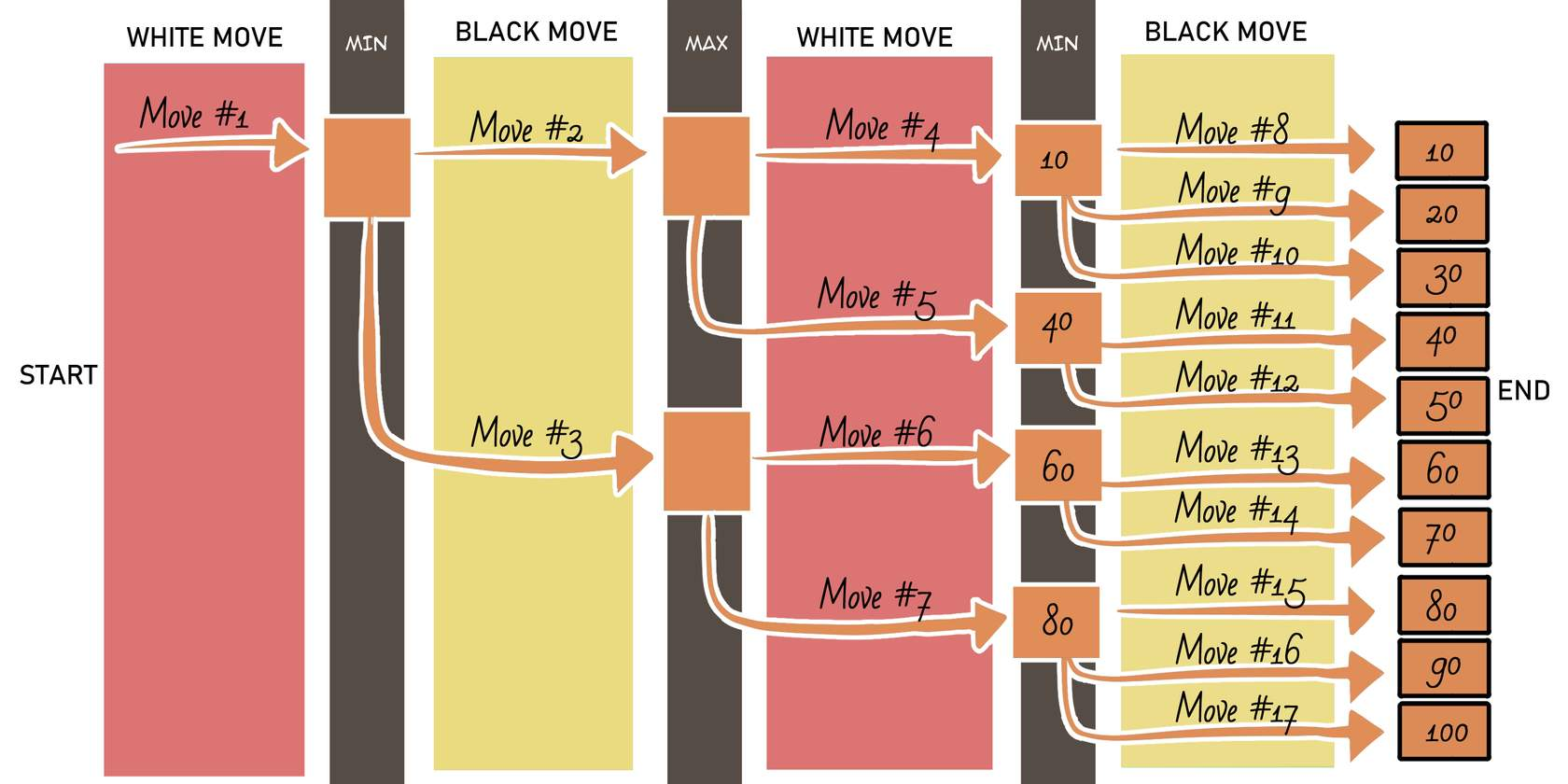### Step 4: Maximize the Potential Score

With the player with white pieces having the previous move, use the same technique as you did before, except now finding the highest score to represent the best move.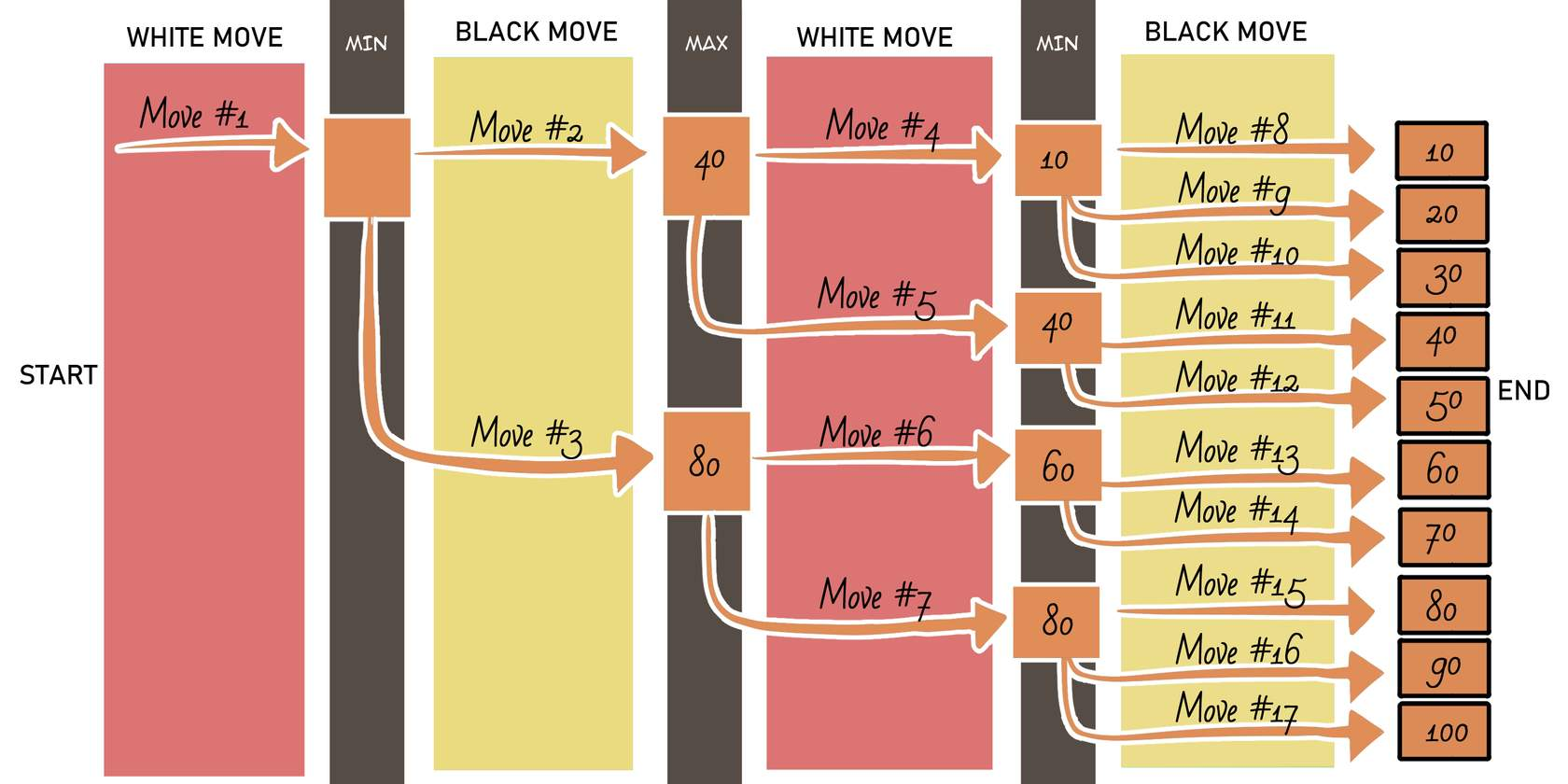### Step 5: Complete the Diagram

Repeat the process to find the final worst-case scenario. Now, by following the arrows to match scores, you can find the most ideal move combination by reading the move labels that are listed on the arrows for the ideal board score.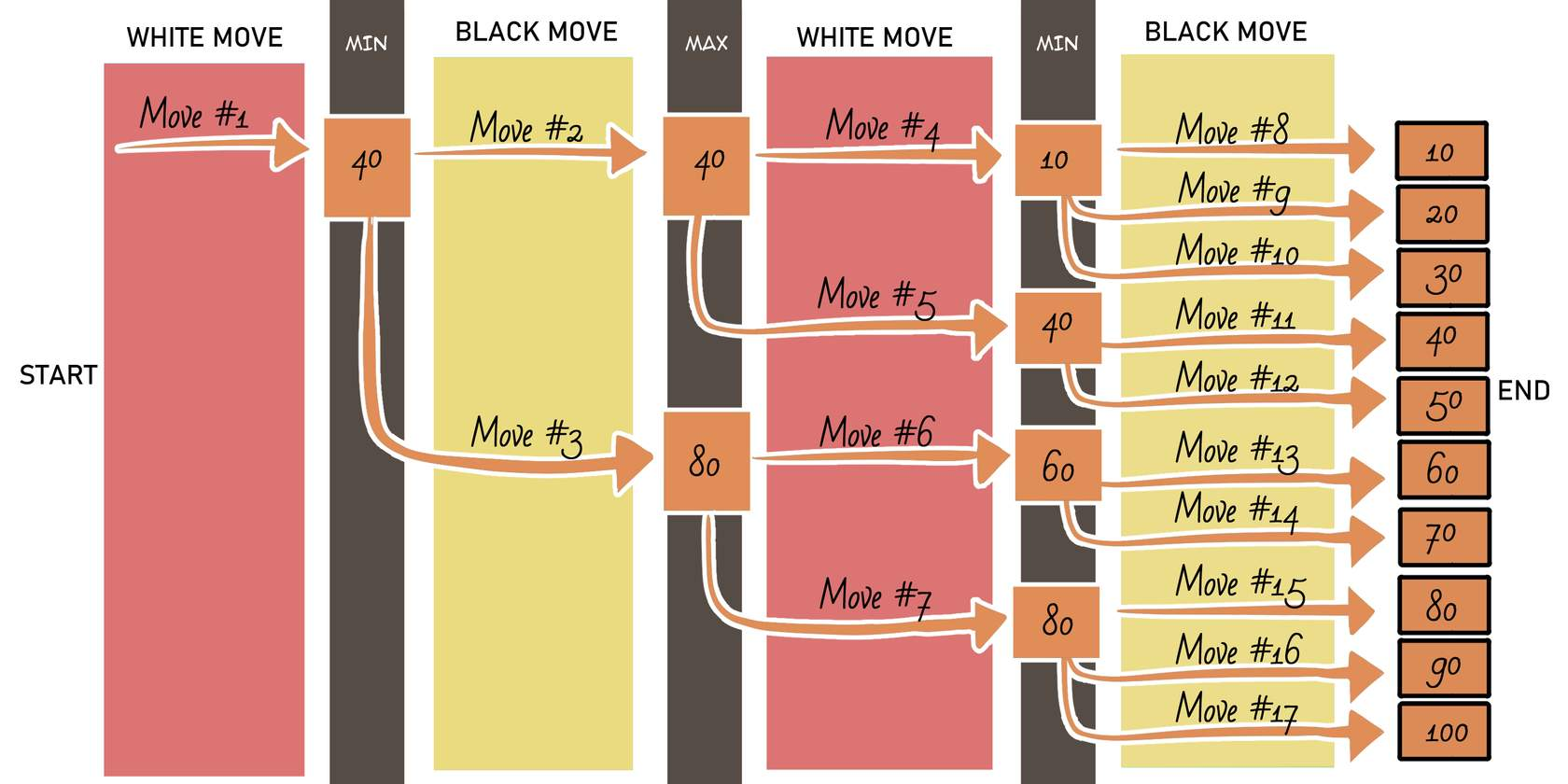The ideal move combination for the example above is move #1 > move #2 > move #5 > move #11.

## Negamax Variation

In a typical minimax algorithm, you would need a function to evaluate the score for the original player and a separate function to evaluate the score for the opponent. However, in chess, the scores for both players are related because chess is a zero-sum game.

In a zero-sum game, the total sum of each outcome is always zero because, in order for a player to gain points, another player must lose the exact same number of points.

Due to the relation between points for players, the negamax algorithm is optimized by using the same function for the original player and the opponent, and only negating the total point value found when evaluating for the opponent.Ryan has been in EdTech and with iD Tech for 13 years—building experience, expertise, and knowledge in all things coding, game development, college prep, STEM, and more. He earned his MBA from Santa Clara University after obtaining his Bachelor’s degree from Arizona State. Connect on LinkedIn

Featured Posts

Categories

Authors